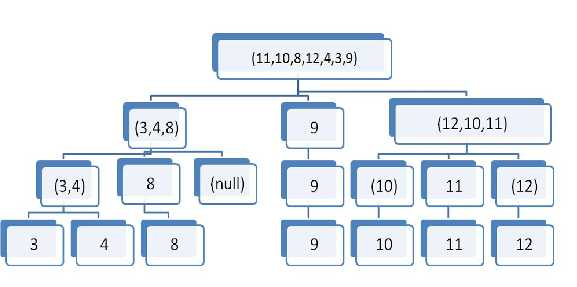DIVIDE AND CONQUER METHOD EXAMPLE

Quick sort is the best example of the divide and conquer technique, so let's go through it once. Please pay attention to each and every word, as each has its own importance in this lesson.
Quick sort

Quick sort was discovered by Tony Hoare in 1962. In this algorithm, the hard work is splitting the array into subsets so that merging the final result is trivial.

1. Choose a pivot element (it can be any element but preferably) from the array.
2. Split the array into three sub-arrays containing the items less than the pivot, the pivot itself, and the items bigger than the pivot.
3. Recursively calling (quick sort) function on the first and last sub-array.

This is exactly what we do:

We put the pivot element on its right position and then again divide the array into two sub-arrays with reference to that pivot element. The newly generated sub-arrays will have their own new pivot elements, the choice of the pivot element is completely user oriented. And then again through the recursive mechanism, these sub-arrays are called in which again their pivot elements are put on their respective position and that array is further divided again. This process continues until we have a single element left in the sub-arrays, which is in itself in its right position.

Let me present a pictorial example to explain quick sort.

We have to sort a given list (11,10,8,12,4,3,9).

So the different steps that comes while under the quick sort algorithm that use the divide and conquer technique is as follows.At the last stage we can see that the given list is sorted.

Here's a more formal specification of the quick sort algorithm. The separate Partition subroutine takes the original position of the pivot element as input and returns the post-partition pivot position as output.
Quicksort(A, p, r):
{
if p < r:
q = Partition(A, p, r) // calling partion function which returns the bifurcation point
Quicksort(A, p, q) // calling quicksort for the first array
Quicksort(A, q+1, r) // calling quicksort for the second array

}

Partition(A, p, r):
{

x = A[p] // considering the first element to ba as the pivot element
i = p - 1
j = r + 1

while 1:
j = j - 1
while A[j] <= x:
j = j - 1

i = i + 1
while A[i] >= x:
j = j - 1

if i < j:

swap(A[i], A[j])
else:
return j

}

In the above code of (Quick sort) we are inputting an array containing the numbers which are to be sort with 'n' as the total number of the numbers present in it. The function (Partition) returns a position about which that array/sub-array is to be partitioned again, this returned value is again used in the recursive calls made by the function (Quick sort).

Let's see the complexity expression for Quick sort

CASE BEST CASE
AVERAGE CASE
WORST CASE
COMPLEXITY O(n logn) O(n logn) O(square of n)
The worst case is one, when the provided list is already sorted.Think about that for some time. If you are unable to find it then click here.

And in order to remove this problem with quick sort, a new sorting called Randomized Quick sort is made, and the only difference is that the pivot element is chosen randomly rather than selecting the same one position of the array as the pivot element.

We will deal with Quick sort in great detail in coming lessons.
Let's see a little about solving the recurrence problems in the next sub-unit after this. I believe it will prove to useful for the coming lessons.

Non-profit Tax ID # 203478467